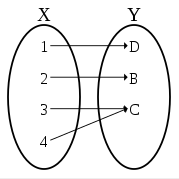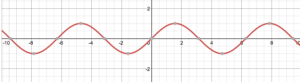# Many to One Function

A many to one function is where several members of the domain map to the same member of the range. Another way of saying this is that different inputs can give the same output.

A one to one function, where distinctness is preserved and every input is matched with a unique output, is called an injection. So a many to one function is not injective.

These mappings don’t have well defined inverses, because knowing the output of the function doesn’t always tell us what the input is.A many to one mapping. Although some members of the domain are mapped one-to-one to distinct members of the range (x = 1 and x = 2), others (x = 3 and x = 42) share the same output (y = C).

## Examples of a Many to One Function

Periodic functions, which repeat at well-defined intervals, are always many-to-one. The trigonometric functions are examples of this; for example, take the function f(x) = sin x. You can prove it is many to one by noting that sin x = sin (2 π + x) = sin (4 π + x), etc., or by noting that when you graph the function, you can draw a straight horizontal line that intersects it many times.In fact, any graphed mapping which a horizontal straight line intersects more than once is a many to one mapping. Since a function always satisfies the vertical line test (no vertical line can intersect more than once), a many to one function is one which any vertical line intersects no more than once but some horizontal line intersects more than once.

## References

Pilkington, Annette. Math 1056 Lecture Notes: Lecture 1. University of Notre Dame Math Department. Retrieved from https://www3.nd.edu/~apilking/Math10560/Lectures/1.%20Inverse%20Functions.pdf. on June 16, 2019
Smith, Ken. Introduction to Functions. Precalculus Lecture Notes. Sam Houston State University Math Department. Retrieved from https://www.shsu.edu/~kws006/Precalculus/1.1_Function_Definition_files/S%26Z%201.3-1.5.pdf on June 16, 2019

CITE THIS AS:
Stephanie Glen. "Many to One Function" From StatisticsHowTo.com: Elementary Statistics for the rest of us! https://www.statisticshowto.com/many-to-one-function/